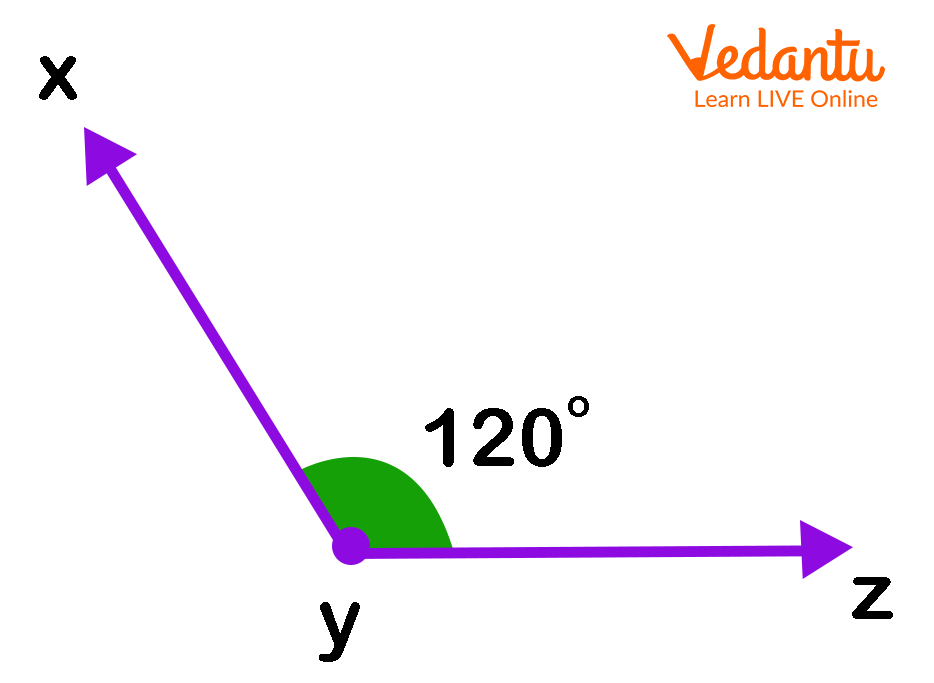Courses
Courses for Kids
Free study material
Free LIVE classes
More

# What is an Obtuse Angle?LIVE
Join Vedantu’s FREE Mastercalss

## Angle Definition

Before we answer the question, let’s understand what an angle is. An angle is a fundamental part of geometry that has been used as an important aspect of architecture and engineering for a long time. We use them to measure changes in the path of motion of ships, stars, airplanes, etc.

But, how do they form?

Angles are formed when two rays or lines intersect at a point. There are different types of Angles in geometry. In this article, we will discuss Obtuse Angles.

## Obtuse Angle: Definition

A simple definition of an obtuse angle is “an angle which is greater than 90o but less than 180o.” Or, we can also say that “it is the angle formed when a ray rotates between 90o and 180o around a point.”

During the day, we can see a clock forming many obtuse angle degrees between the minute hand and the hour hand. Let us understand more about the obtuse angles and their properties.

## Obtuse Angle PicObtuse angle measuring 120o.

## Obtuse Angles in Real Life

Below are some real-life examples of obtuse angles.Obtuse Angle Pictorial representation

If we look up the sofa or other objects and the highlighted angles, then we observe that the angle formed is greater than the right angle, i.e., 90o but less than the straight angle, i.e., 180o. So, these objects are examples of obtuse angles.

## Properties of an Obtuse Angle

• The angles always range from 90o and 180o. It means an obtuse angle is greater than 90 degrees and smaller than a straight angle that is 180 degrees.

• This angle will resemble a quarter of the circle but not half of the circle.

• If we divide the circle into four pieces, an obtuse angle will occupy between $\frac{1}{4}$ and $\frac{1}{2}$ of a circle.

• In an obtuse triangle, there will always be an obtuse angle.

## Conclusion

An obtuse angle is defined as 'an angle whose measure is larger than 90° but less than 180o'. A protractor can be used to measure or draw an obtuse angle. This is a helpful tool that students will learn to use in geometry lessons.

To learn more geometrical concepts or any other mathematical concepts, explore our website.

Last updated date: 28th Sep 2023
Total views: 135.9k
Views today: 3.35k

## FAQs on What is an Obtuse Angle?

1. How to determine if a triangle is obtuse?

The triangle is acute if the sum of the squares of the two shorter sides is greater than the square of the longest side. The triangle is obtuse if the sum of the squares of the two shorter sides of a triangle is less than the square of the longest side.

2. What is the obtuse angle triangle?

When one of the triangle's angles is greater than 90 degrees, it is called an obtuse angle. Obtuse triangles include isosceles and scalene triangles. It is not possible for equilateral triangles to be obtuse as all angles measure 60 degrees. The angle opposite the obtuse angle is the triangle's longest side.